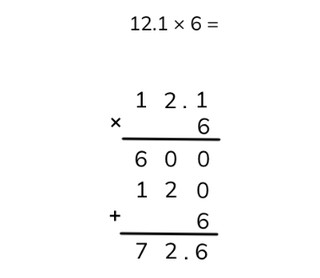Partial products algorithm with one decimal number

# Partial products algorithm with one decimal number

Students learn to calculate partial products with one decimal number.8,000 schools use Gynzy92,000 teachers use Gynzy1,600,000 students use Gynzy

## General

Students learn partial products multiplication with a decimal number.

## Standards

CCSS.Math.Content.5.NBT.B.7

## Learning objective

Students will be able to calculate partial products with decimal numbers.

## Introduction

Spin the wheel and have a student name a number to 100. Fill in the numbers on the orange and blue line. Then the students solve the problem.

## Instruction

Explain that with partial products multiplication you put the numbers of the problem under one another. Next you can multiply them by calculating from left to right. Explain that you put the decimal number of the problem on top and the other number of the problem underneath that. With the partial products algorithm you work from left to right. Explain how you can multiply a problem using partial products with a decimal number with one decimal place. You start by imagining that the decimal point is not there. In this way, you multiply with whole numbers. You put all the answers in the chart and give the intermediate problems. If you add up the numbers from the chart, you know what the answer is. You put this answer at the bottom of the chart. Then you have to put the decimal point back in. Check in the problem how many digits there are behind the decimal point, that is how many should also be behind the decimal point in the answer. Next there are problems in a chart, each one with a different row marked in yellow. Ask the students if they know which intermediate problem belongs with each of the colored rows. This is how you can check if students know which steps they need to follow. Next you show that you multiply partial products with a decimal number with two decimal places in the same way as with one decimal place. Have the students practice this, by calculating a problem using partial products. Drag the correct numbers to the chart. After this, you show how you can use partial products to multiply a problem with a decimal number with three decimal places. Also have the students practice this by multiplying using partial products. Then you explain that with the partial products algorithm, when you multiply a number with 0 then you don't have to write down the answer. When you multiply by 0 the answer is always 0. Next you show a chart, for which the intermediate steps must be filled in. Have the students write these numbers down and hold them up, so that you can check them.

Check whether the students can calculate partial products with one decimal number through the following questions/exercise:
- Why is it useful to be able to multiply with partial products?
- Where do you always start when calculating partial products?
- Solve this problem using partial products: 30.271 × 5

## Quiz

The students test their understanding of calculating partial products with one decimal number through ten exercises. In these exercises they must solve multiplication problems with decimal numbers with one, two, or three decimal places.

## Closing

You discuss once again with the students that it is important to be able to multiply using partial products with one decimal number, because that is how you can solve problems in simple steps. Check if the students know that when they multiply partial products they must calculate from left to right. Have the students come up with problems on their own by dragging numbers to the chart.

## Teaching tips

When students have difficulty with partial products multiplication, you can repeat the meaning of T H T O t h t and how you put the numbers into a chart. Write out the intermediate problems. You may also label the tens, ones, tenths, hundredths, and thousandths, so that it is obvious what is being multiplied together.

### The online teaching platform for interactive whiteboards and displays in schools

• Save time building lessons

• Manage the classroom more efficiently

• Increase student engagement